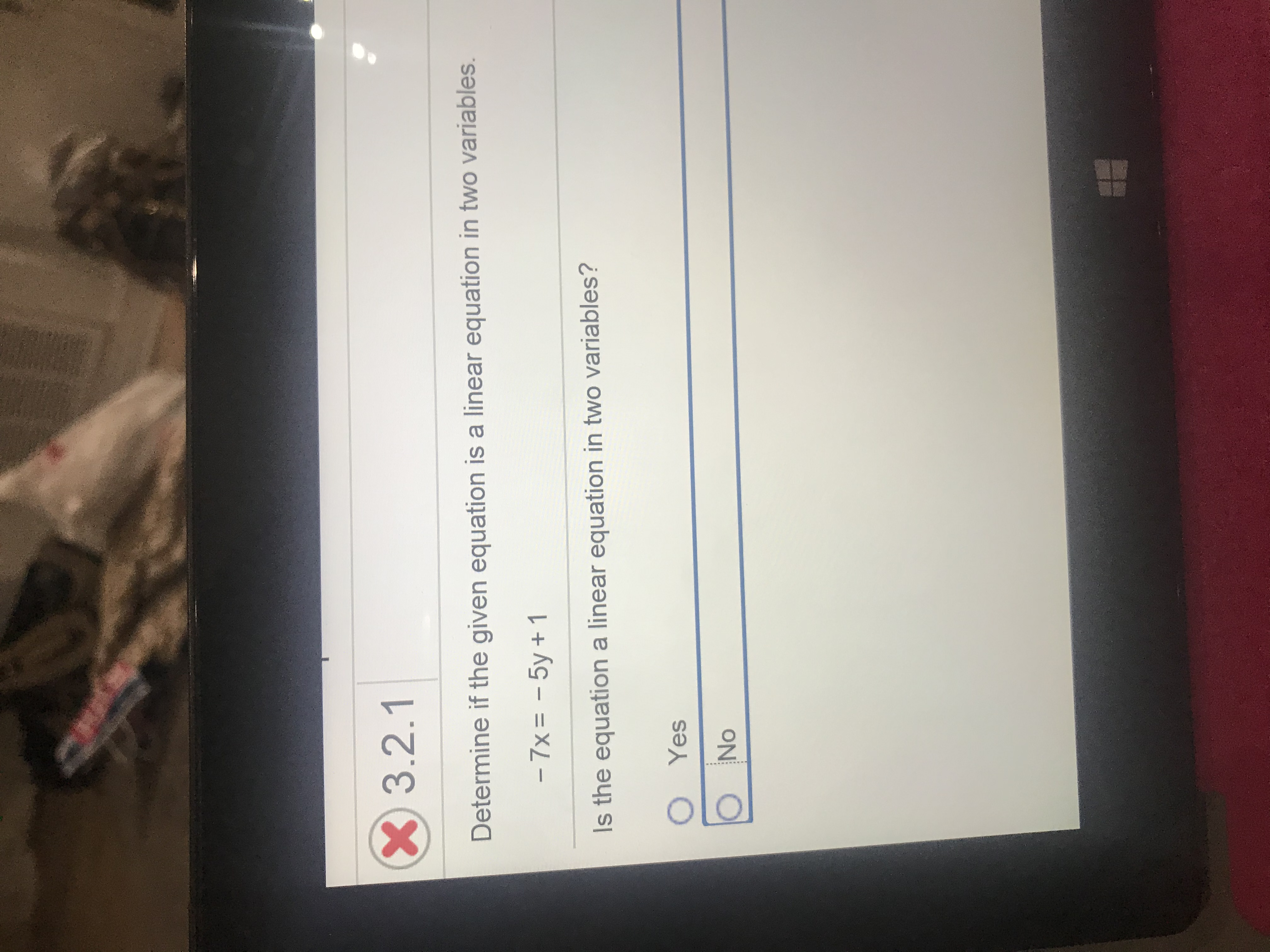# X3.2.1Determine if the given equation is a linear equation in two variables.- 7x = - 5y +1Is the equation a linear equation in two variables?O YesNo

Question
2 viewshelp_outlineImage TranscriptioncloseX3.2.1 Determine if the given equation is a linear equation in two variables. - 7x = - 5y +1 Is the equation a linear equation in two variables? O Yes No fullscreen
check_circle

Step 1

According to Linear equation in two variables definition:

An equation which is in the form of mx + ny + p = 0,

Where m, n, and p are real numbers and a, b not equal to zero.

The solution fo...

### Want to see the full answer?

See Solution

#### Want to see this answer and more?

Solutions are written by subject experts who are available 24/7. Questions are typically answered within 1 hour.*

See Solution
*Response times may vary by subject and question.
Tagged in